# Number systems for humans, Simpsons and computers

This is an introduction to the many ways of counting.

## How do humans count?

When you first learned to count, you probably used your fingers.  Some people start with their pointer finger, or even their pinky, but here in France we start with our thumb as shown below: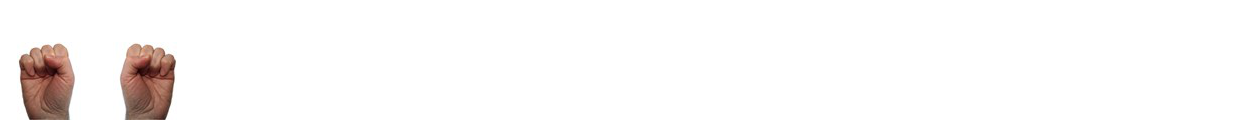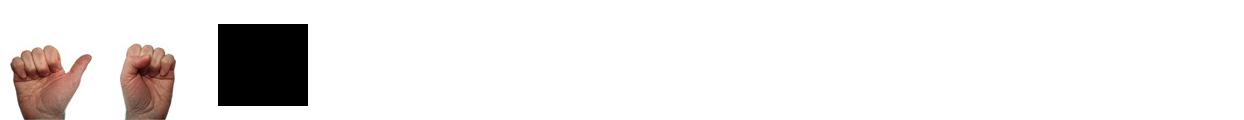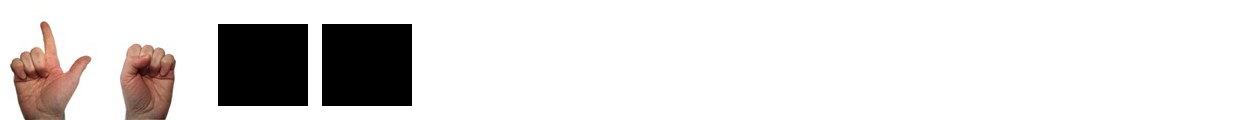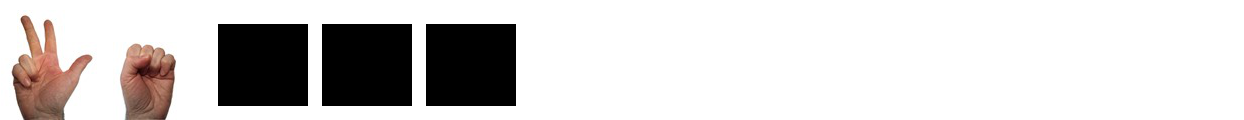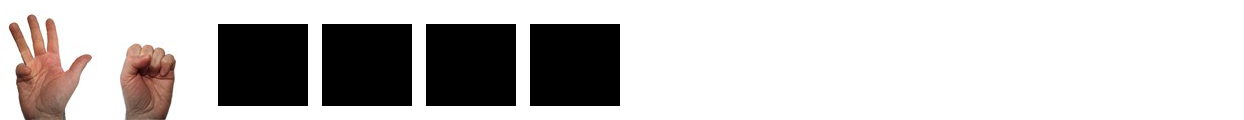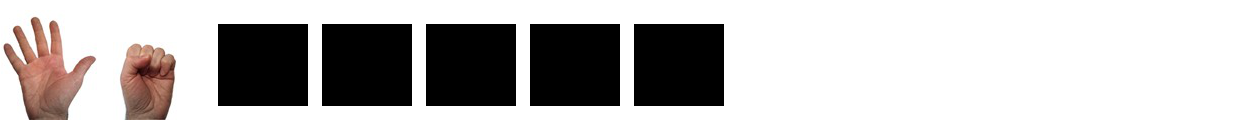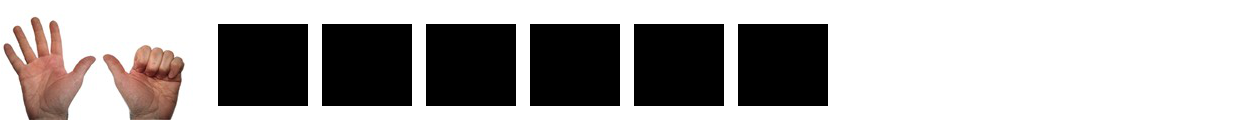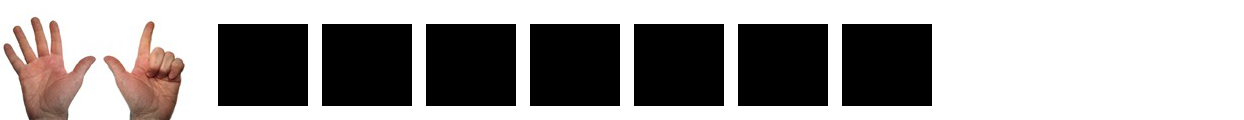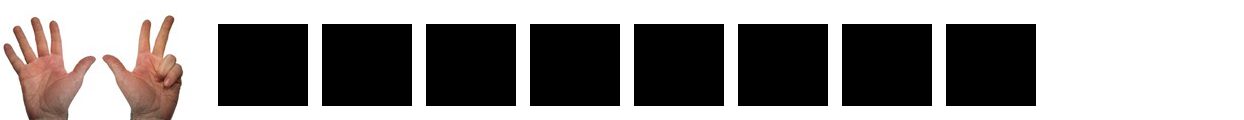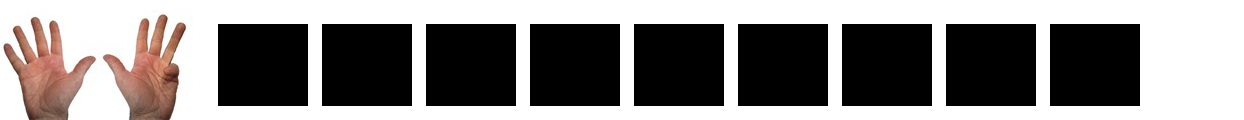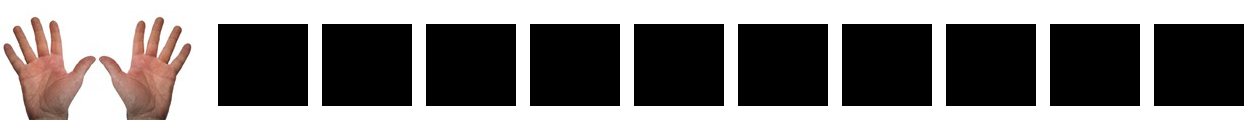Most people have ten fingers, so our number system is based on ten. When we write numbers, we use ten unique symbols called digits:

0 1 2 3 4 5 6 7 8 9

Using these ten symbols, we can write any number.  The key is that after we pass nine, we start writing two digits, where the first digit is automatically assumed to be multiplied by ten.  When we write
“26”, we know that we have 2 times ten plus 6: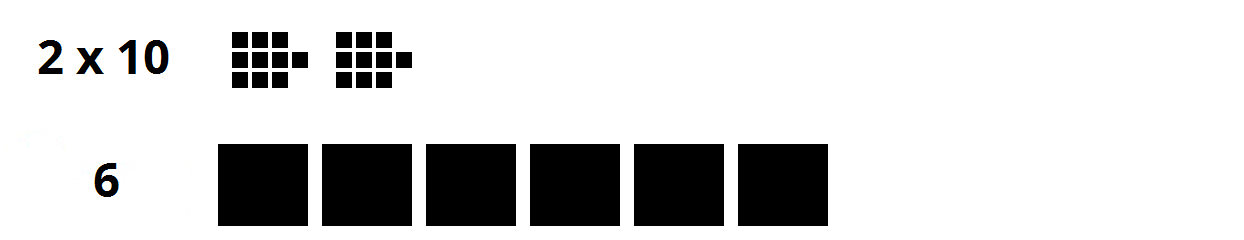After we pass ninety-nine, we start using three digits.  The first digit represents the number of hundreds (ten times ten), the second digit represents the number of tens, the third digit represents the number of ones.  When we write “256”, we know that we have 2 times one hundred plus 5 times ten plus 6: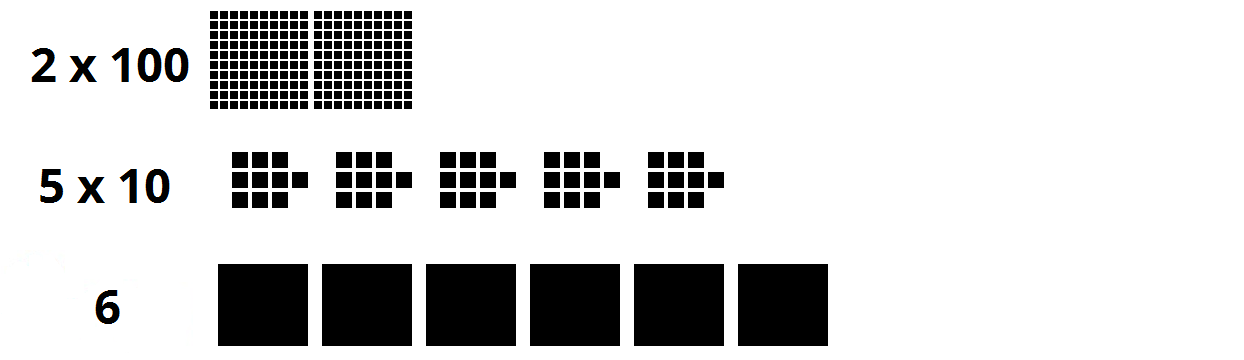After the hundreds digit, we add a thousands digit (ten times ten times ten) and then a ten thousands digit (ten times ten times ten times ten).  I could keep going, but I think you get the point.  We have ten fingers, ten unique digits, and each time we get to our limit, we add another digit that is ten times greater than the last.  That’s a lot of tens.

Computers don’t have fingers.  To keep things straight, whenever we write about human counting from now on, we’ll put a little “10” to the lower right.  So when a human says “twenty-six”, we’ll write “2610”.  When a human says “two hundred fifty-six”, we’ll write “25610”. The little “10” doesn’t mean multiply. It’s just a way of reminding ourselves that we count based on ten.

The human number system is called “decimal”, which means based on ten. When engineers talk about “human counting numbers”, they say “base ten”:

110 is pronounced “one base ten”.
2610 is pronounced “twenty-six base ten”.
25610 is pronounced “two hundred fifty six base ten”.

## How do the Simpsons count?

If you have watched the animated TV series “The Simpsons”, you may have noticed that each of their hands has four fingers.  The Simpsons seem to do math based on decimal, probably because their creator, Matt Groening, is a human.  But what if they used a system based on their eight fingers?  They would only use eight unique symbols for their digits, which means they would never use “8” or “9”.  They would only use these digits:

0 1 2 3 4 5 6 7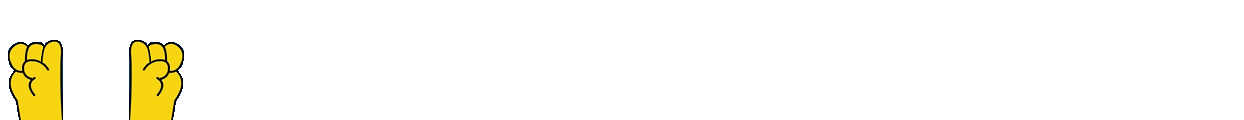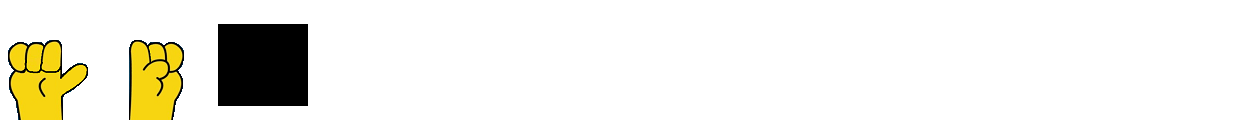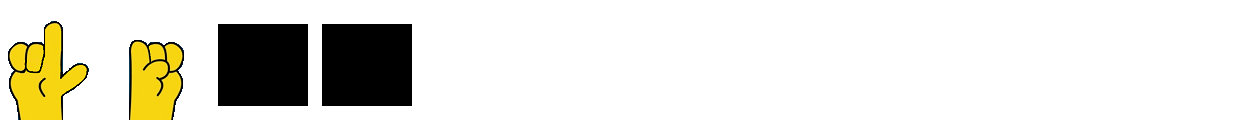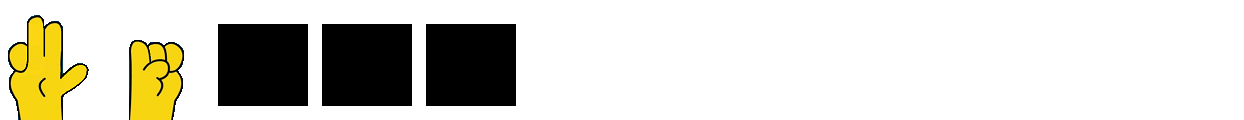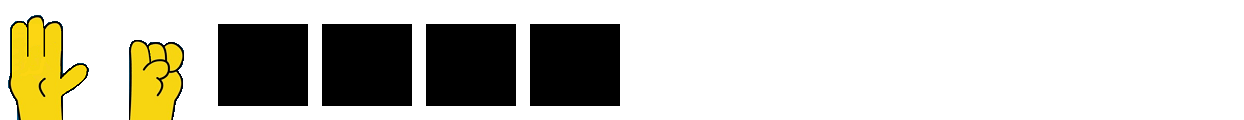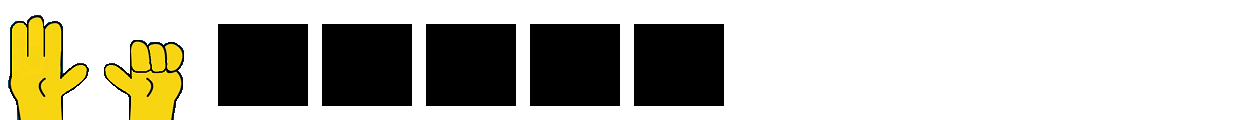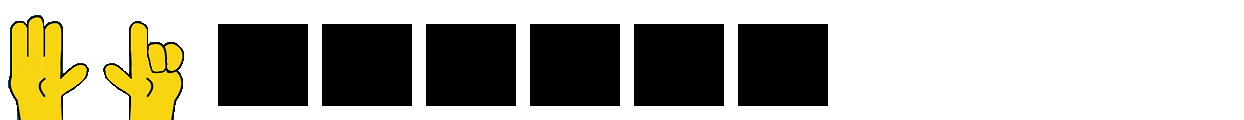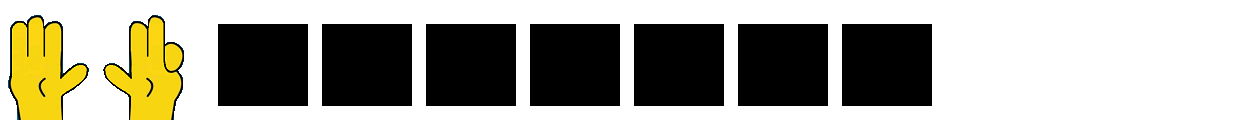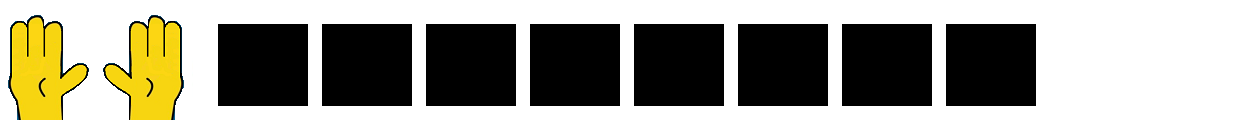Using these eight symbols, the Simpsons still can write any number, but it gets a little more complicated.  Now, after they pass seven, they have to start writing two digits, where the first digit is automatically assumed to be multiplied by eight.  Their number system would be “base 8”.  Base 8 is also called octal.  To help you remember “octal”, think of an octopus that has eight tentacles.  When we write a number based on octal, we put a little “8” to the lower right.

When the Simpsons write “268”, they mean 2 times eight plus 6: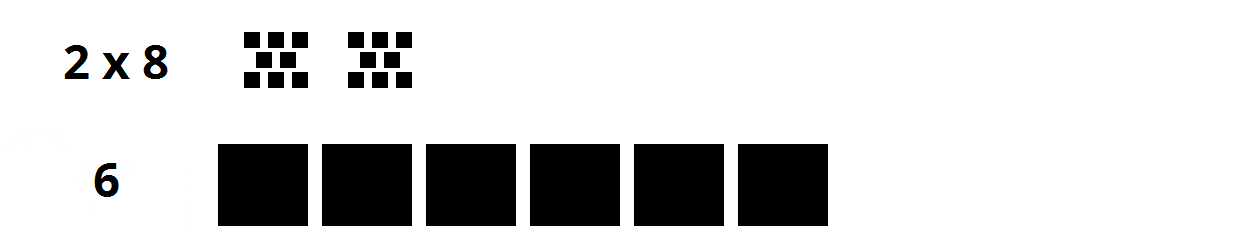After the Simpsons pass “778”, they start using three digits.  The first digit represents the number of eight times eight (6410), the second digit represents the number of eights, the third digit represents the number of ones.  When the Simpsons wrote “2568”, they mean 2 times 6410 plus 5 times eight plus 6: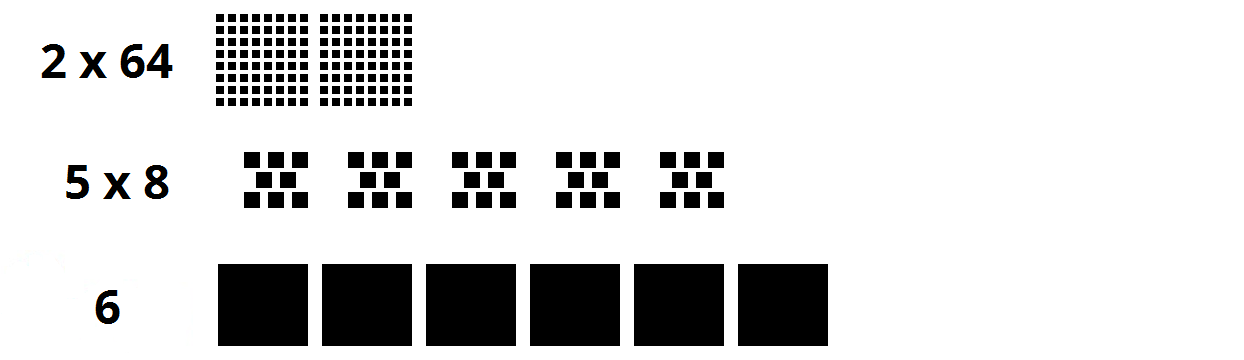If you count the blocks in all of the examples above, you will see that 2610 does NOT equal 268, and 25610 does NOT equal 2568. However, it’s easy fairly easy to convert to base 10 (human counting) from any other base. You just have to multiply and add as shown in the examples below:

268 = (2 x 8) + 6 = 2210

2568 = (2 x 8 x 8) + (5 x 8) + 6 = 17410

410758 = (4 x 8 x 8 x 8 x 8) + (1 x 8 x 8 x 8) + (0 x 8 x 8) + (7 x 8) + 5 = 1695710 (Don’t forget the zero when you multiply!)

188 = ???? There is no answer. This number doesn’t exist in base 8, because the digits only go up to 7.

908 = ???? There is no answer. This number doesn’t exist in base 8, because the digits only go up to 7.

So, if most people had eight fingers, we would all use the octal number system. The digits “8” and “9” wouldn’t exist. As we added digits to the left of a number, we would multiply by an extra 8 each time.

I didn’t explain how to convert from base 8 to base 10 yet.  This is a little more difficult and will be discussed later.

## How do computers count?

Computers don’t have fingers.  Computers today only know about “0” and “1”.  Computers can only count up to one before adding another digit.  Computers only know these symbols for their digits:

0 1

Computers don’t know ANY of these digits (barred for emphasis):

2 3 4 5 6 7 8 9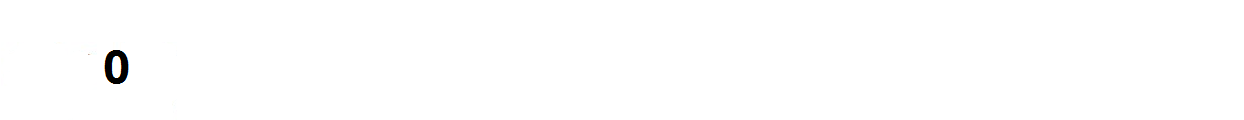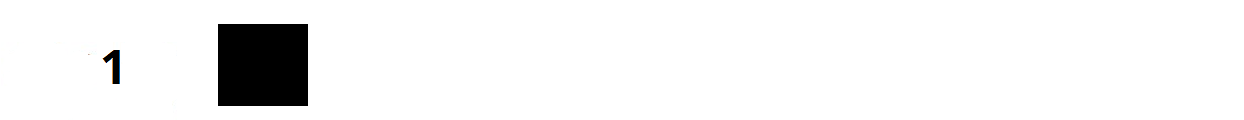With just two symbols (0 and 1), the computer counts in base 2.  Base 2 is also called the binary number system.    To help you remember “binary”, think of a bicycle that has two wheels.  When we write a number based on binary, we put a little “2” to the lower right.  A computer can count 0, then 1, and then it must add a digit.  With two digits, the first digit is automatically assumed to be multiplied by two.

When a computer thinks of “112”, it means 1 times two plus 1: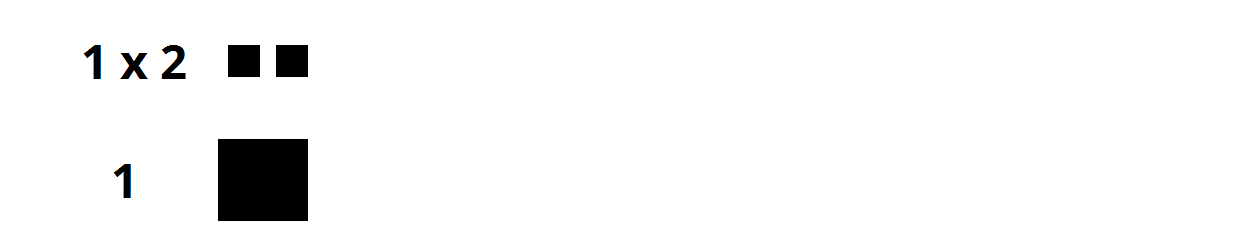After a computer passes “112”, it starts using three digits.  The first digit represents the number of two times two (410), the second digit represents the number of twos, the third digit represents the number of ones.  When a computer thinks of “1012”, it means 1 times 410 plus 0 times two plus 1: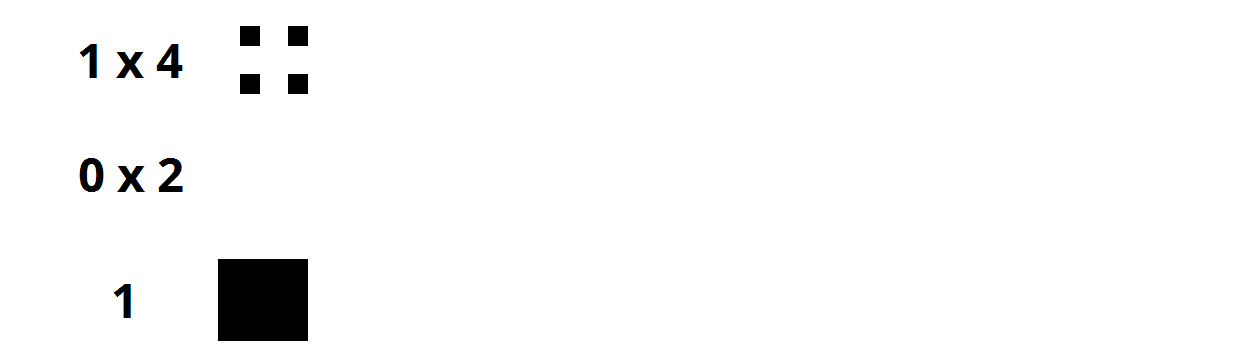When you count in binary, you end up with long strings of digits. The digits in binary (“0” or “1”) are called “bits”. Bits are binary digits. You may need to know how many bits a number uses. You just have to count the number of digits in the number:

How many bits are in 1012? 3 bits

How many bits are in 110100012? 8 bits

You also need to be able to convert from binary to decimal:

11012 = (1 x 2 x 2 x 2) + (1 x 2 x 2) + (0 x 2) + 1 = 1310

That’s all I’ll write about binary for now, but a good understanding of binary numbers is essential for learning how computers work.

## Why are there zeros in front of that number?

Have you heard of 007?  Why do they put those zeros in front of James Bond’s code name.  It’s a place holder to indicate that there are many more spies (at least one hundred based on those zeros).

Computers like to add preceding zeros.  When we store a number in a computer, we reserve space for it’s maximum value.  To show how much space we have reserved, we often “pad” the number with preceding zeros.  This is particularly true for binary numbers.

For example, if we have a counter for the number of children in a family, we could say the current value is three children, but that it could increase to a maximum of fifteen children.  To show that we have reserved extra space, we would write three as 00112. This shows that we could go up to fifteen or 11112.

For binary, we have names for the number of bits reserved.  Here’s a table to show the most common ones:

Name# of bitsmaximum binary valuemax decimal value
bit111
nibble4111115
byte811111111255
word16111111111111111165535
double word32111111111111111111111111111111114294967295
Common names for groups of bits

This entry was posted in Uncategorized on .